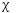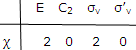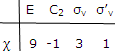Molecular Symmetry Types

The number of molecular orbitals and molecular motions of each symmetry type can be deduced.

Let us continue to use the C2v point group and the H2O molecule to illustrate how the procedure developed in preceding sections can be used to deduce the symmetry of molecular properties.

Bond orbitals to molecular orbitals: suppose that the electrons in one bond of an H2O molecule are described by a bond Ø1 and those in the other bond by Ø2. These bond orbitals can be used to construct molecular orbitals. Each molecular orbital must transform according to one of the symmetry species of the C2v point group, that to which the H2O molecule belongs.

The transformation matrices that describe the effect of the various symmetry operations on the two bond orbitals can be seen.

The characters of the representation provided by these transformation matrices are the sums of the diagonal elements. If we writeto represent the characters, we haveAccording to the deduction these are also the characters that would have been obtained if we had used molecular orbitals as a basis. But the characters for each molecular orbital must be drawn from those for the irreducible representation of the point group.=Ai +Bi

Thus we have discovered that the molecular orbitals, the eigenfunctions for the H2O molecule that are constructed from orbitals along the individual bonds, must be of the symmetry types A1 and B1.

A convenient way to obtain characters: the transformation matrices do not have to be written to obtain the characters 2, 0, 2, 0 shown above. A diagonal element occurs only if the bond is turned into itself or in other cases turned into the opposite of itself. Bonds (or vectors or orbitals) shifted from one to another equivalent atom do not degenerate any diagonal elements. Thus, with for each symmetry operation we write a contribution of +1 for each unshifted bond orbital. The result for E is 2, for C2 is 0, for σv is 2, and for σ'v is 0. These are the same characters as we obtained earlier by working out the transformation matrices.

Translations, rotations and vibrations: now let us see whether we can classify the overall translational and rotational motions and the vibrational motions of the H2O molecule according to symmetry. We begin as in the analysis of the number of degrees of freedom by treating these motions in terms of the three displacement coordinates on each atom. These 3n vectors provide a basis on which the symmetry operation of the C2v group, to which the H2O molecule belongs, can act.

We need not work out the 9 × 9 transformations matrices that describe the effect of EC2σvand σ'v on the nine displacement vectors. We need only deduce the characters i.e. the sum of the diagonal elements, of these matrices.

An entry occurs along the diagonal of a transformation matrix for an atom cannot exchange places with another atom as a result of the symmetry operation. Thus for each exchange places operations effect of each symmetry operation will be to leave each of these vectors unchanged or to reverse them. In the former case a + 1 will be contributed to the diagonal terms; in the latter case a - 1 will be contributed. Inspection of the effect of the symmetry operations on the nine displacement vectors that provide a basis for the translations, rotations, and vibrations of the molecule givesNow, in principle, a change in coordinates could be found such that the nine displacement coordinates would combine to give coordinates for the three translational motions and the three rotational motions of the molecule as a whole and the three vibrational motions of the atoms of the molecule. (Such a transformation was worked through for diatomic molecules.

The transformation matrices that describe the effects of the symmetry operations on these new displacement sets would be different from those describing the effects on the displacement vectors. But the sum of the diagonal elements of these transformation matrices would be unaffected. Thus we can treat the set of numbers 9, -1, 3, 1 as being the net characters for the three translational, three rotational, and three vibrational motions.

We can express the result by writing= 3A1 +A2 + 3B1 + 2B2

#### Related Questions in Chemistry

• ##### Q :Coordination number of a cation The

The coordination number of a cation engaging a tetrahedral hole is: (a) 6  (b) 8  (c) 12  (d) 4 Answer: (d) The co-ordination number of a cation occupying a tetrahedral hole is 4.

• ##### Q :Haloalkanes define primary secondary

define primary secondary and tertiary alkyl halides with examples

• ##### Q :Oxoacids of halogens Why oxidising

Why oxidising character of oxoacids of halogens decreases as oxidation number increases?

• ##### Q :Surface Tension Vapour Pressure The

The vapor pressure of small liquid drops depends on the drop size. Although the surface properties of a liquid are different from those of the bulk liquid, the special surface properties can be ignored except in a few situations. One is the case in which a liquid is dispersed into fine dr

• ##### Q :How haloalkanes are prepared from

This is the common method for preparing haloalkanes in laboratory. Alcohols can be converted to haloalkanes by substitution of - OH group with a halogen atom. Different reagents can be used to get haloa

• ##### Q :Molal elevation constant of water The

The boiling point of 0.1 molal aqueous solution of urea is 100.18oC  at 1 atm. The molal elevation constant of water is: (a) 1.8    (b) 0.18   (c) 18    (d) 18.6Answer: (a) Kb

• ##### Q :Distribution law Help me to go through

Help me to go through this problem. The distribution law is applied for the distribution of basic acid between : (a) Water and ethyl alcohol (b) Water and amyl alcohol (c) Water and sulphuric acid (d) Water and liquor ammonia

• ##### Q :Mole fraction of hydrogen Give me

Give me answer of this question. In a mixture of 1 gm H2 and 8 gm O2 , the mole fraction of hydrogen is: (a) 0.667 (b) 0.5 (c) 0.33 (d) None of these

• ##### Q :Problem based on molarity Select the

Select the right answer of the question. If 18 gm of glucose (C6H12O6) is present in 1000 gm of an aqueous solution of glucose, it is said to be: (a)1 molal (b)1.1 molal (c)0.5 molal (d)0.1 molal

• ##### Q :Electrochemistry ( electrolysis of

1. Define Faraday's first law of electrolysis 2. define Faraday's second law of electrolysis